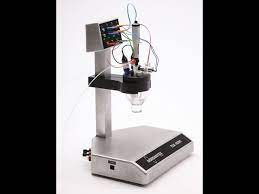## POLAROGRAPHY-AN ELECTRO-ANALYTICAL TECHNIQUEPolarography is an electroanalytical technique that measures the current flowing between two electrodes in the solution (in the presence of gradually increasing applied voltage) to determine the concentration of solute and its nature respectively. It is a type of voltammetry where the working electrode is a dropping mercury electrode (DME) or a static mercury drop electrode (SMDE), which are useful because they have a wide cathodic range and renewable surface.

Voltammetry is a category of electroanalytical methods where the information about the analyte is obtained by measuring current as the potential is varied. The analytical data for a voltammetric experiment is depicted in the form of a voltammogram (a polarogram in case of polarography) which plots the current produced by the electrolyte vs. the potential of the working electrode.The current flowing through the working electrode is made up of two components:

1. Faradic Current (iF) which is based on the oxidation and reduction of the analyte and contributes to the useful signal.

2. Capacitive Current(iC) which is generated by charging and discharging of the electrochemical double layer on the surface of the working electrode and contributes to the unwanted interference component (noise) in the signal.

Polarography is a voltammetric measurement whose response is determined by only diffusion mass transport. The simple principle of polarography is the study of solutions or of electrode processes by means of electrolysis with two electrodes, one polarizable and one unpolarizable, the former formed by mercury regularly dropping from a capillary tube. Polarography is a specific type of measurement that falls into the general category of linear-sweep voltammetry where the electrode potential is altered in a linear fashion from the initial potential to the final potential. As a linear sweep method controlled by convection/diffusion mass transport, the current vs. potential response of a polarographic experiment has the typical sigmoidal shape. What makes polarography different from other linear sweep voltammetry measurements is that polarography makes use of the dropping mercury electrode (DME) or the static mercury drop electrode.

A plot of the current vs. potential in a polarography experiment shows the current oscillations corresponding to the drops of Hg falling from the capillary. If one connected the maximum current of each drop, a sigmoidal shape would result. The limiting current (the plateau on the sigmoid), called the diffusion current because diffusion is the principal contribution to the flux of electroactive material at this point of the Hg drop life.

Limitations

There are limitations in particular for the classical polarography experiment for quantitative analytical measurements. Because the current is continuously measured during the growth of the Hg drop, there is a substantial contribution from capacitive current. As the Hg flows from the capillary end, there is initially a large increase in the surface area. As a consequence, the initial current is dominated by capacitive effects as charging of the rapidly increasing interface occurs. Toward the end of the drop life, there is little change in the surface area which diminishes the contribution of capacitance changes to the total current. At the same time, any redox process which occurs will result in faradaic current that decays approximately as the square root of time (due to the increasing dimensions of the Nernst diffusion layer). The exponential decay of the capacitive current is much more rapid than the decay of the faradaic current; hence, the faradaic current is proportionally larger at the end of the drop life. Unfortunately, this process is complicated by the continuously changing potential that is applied to the working electrode (the Hg drop) throughout the experiment. Because the potential is changing during the drop lifetime (assuming typical experimental parameters of a 2 mV/s scan rate and a 4 s drop time, the potential can change by 8 mV from the beginning to the end of the drop), the charging of the interface (capacitive current) has a continuous contribution to the total current, even at the end of the drop when the surface area is not rapidly changing. As such, the typical signal to noise of a polarographic experiment allows detection limits of only approximately 10−5 or 10−6 M.